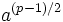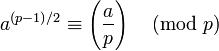# Euler's criterion

## Statement

### In terms of quadratic residues and nonresidues

Supposeis an odd prime number. Consider an integerthat is not zero mod. Then:

•is congruent to either 1 or -1 mod.
•is congruent to 1 modif and only ifis a quadratic residue mod.
•is congruent to -1 modif and only ifis a quadratic nonresidue mod.

### In terms of Legendre symbol

Supposeis an odd prime number. Consider an integerthat is not zero mod. Then:where the expression on the right side is the Legendre symbol, defined to befor a quadratic residue andfor a quadratic nonresidue. Note that the Legendre symbol is the restriction to primes of the Jacobi symbol.